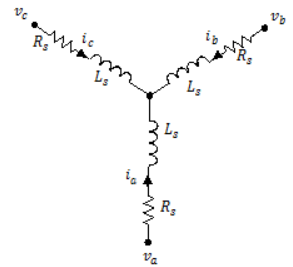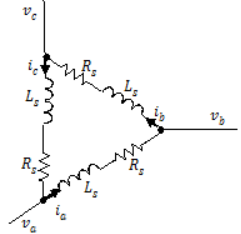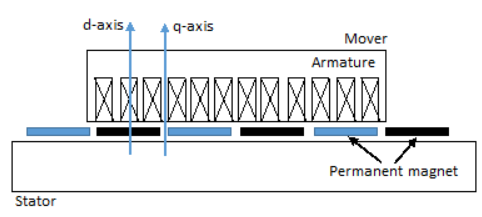# PMLSM

Permanent magnet linear synchronous motor with sinusoidal flux distribution

• Library:
• Simscape / Electrical / Electromechanical / Permanent Magnet

•## Description

The PMLSM block models a permanent magnet linear synchronous motor with a three-phase wye-wound stator. The figure shows the equivalent electrical circuit for the stator windings.You can also model the permanent magnet linear synchronous motor in a delta-wound configuration by setting Winding type to `Delta-wound`.### Motor Construction

This figure shows the motor construction.### Equations

Voltages across the stator windings are defined by:

`$\left[\begin{array}{c}{v}_{a}\\ {v}_{b}\\ {v}_{c}\end{array}\right]=\left[\begin{array}{ccc}{R}_{s}& 0& 0\\ 0& {R}_{s}& 0\\ 0& 0& {R}_{s}\end{array}\right]\left[\begin{array}{c}{i}_{a}\\ {i}_{b}\\ {i}_{c}\end{array}\right]+\left[\begin{array}{c}\frac{d{\psi }_{a}}{dt}\\ \frac{d{\psi }_{b}}{dt}\\ \frac{d{\psi }_{c}}{dt}\end{array}\right],$`

where:

• va, vb, and vc are the individual phase voltages across the stator windings.

• Rs is the equivalent resistance of each stator winding.

• ia, ib, and ic are the currents flowing in the stator windings.

• $\frac{d{\psi }_{a}}{dt},$$\frac{d{\psi }_{b}}{dt},$ and $\frac{d{\psi }_{c}}{dt}$ are the rates of change of magnetic flux in each stator winding.

The permanent magnet and the three windings contribute to the total flux linking each winding. The total flux is defined by:

`$\left[\begin{array}{c}{\psi }_{a}\\ {\psi }_{b}\\ {\psi }_{c}\end{array}\right]=\left[\begin{array}{ccc}{L}_{aa}& {L}_{ab}& {L}_{ac}\\ {L}_{ba}& {L}_{bb}& {L}_{bc}\\ {L}_{ca}& {L}_{cb}& {L}_{cc}\end{array}\right]\left[\begin{array}{c}{i}_{a}\\ {i}_{b}\\ {i}_{c}\end{array}\right]+\left[\begin{array}{c}{\psi }_{am}\\ {\psi }_{bm}\\ {\psi }_{cm}\end{array}\right],$`

where:

• ψa, ψb, and ψc are the total fluxes linking each stator winding.

• Laa, Lbb, and Lcc are the self-inductances of the stator windings.

• Lab, Lac, Lba, Lbc, Lca, and Lcb are the mutual inductances of the stator windings.

• ψam, ψbm, and ψcm are the permanent magnet fluxes linking the stator windings.

The inductances in the stator windings are functions of electrical angle, and are defined by:

`${\theta }_{e}={N}_{p}x+rotor\text{\hspace{0.17em}}offset,$`

`${L}_{aa}={L}_{s}+{L}_{m}\text{cos}\left(2{\theta }_{e}\right)$`

`${L}_{bb}={L}_{s}+{L}_{m}\text{cos}\left(2\left({\theta }_{e}-2\pi /3\right)\right)$`

`${L}_{cc}={L}_{s}+{L}_{m}\text{cos}\left(2\left({\theta }_{e}+2\pi /3\right)\right)$`

`${L}_{ab}={L}_{ba}=-{M}_{s}-{L}_{m}\mathrm{cos}\left(2\left({\theta }_{e}+\pi /6\right)\right)$`

`${L}_{bc}={L}_{cb}=-{M}_{s}-{L}_{m}\mathrm{cos}\left(2\left({\theta }_{e}+\pi /6-2\pi /3\right)\right)$`

and

`${L}_{ca}={L}_{ac}=-{M}_{s}-{L}_{m}\mathrm{cos}\left(2\left({\theta }_{e}+\pi /6+2\pi /3\right)\right)$`

where:

• θe is the electrical angle.

• rotor offset is `0` if you define the rotor electrical angle with respect to the d-axis, or `-pi/2` if you define the rotor electrical angle with respect to the q-axis.

• ${N}_{p}=\frac{\pi }{\tau }$ and τ is the polar pitch.

• x is the distance.

• Ls is the per-phase stator self-inductance. This value is the average self-inductance of each of the stator windings.

• Lm is the stator inductance fluctuation. This value is the fluctuation in self-inductance and mutual inductance with changing angle.

• Ms is the stator mutual inductance. This value is the average mutual inductance between the stator windings.

The permanent magnet flux linking winding a is a maximum when θe = 0° and zero when θe = 90°. Therefore, the linked motor flux is defined by:

`$\left[\begin{array}{c}{\psi }_{am}\\ {\psi }_{bm}\\ {\psi }_{cm}\end{array}\right]=\left[\begin{array}{c}{\psi }_{m}\mathrm{cos}{\theta }_{e}\\ {\psi }_{m}\mathrm{cos}\left({\theta }_{e}-2\pi /3\right)\\ {\psi }_{m}\mathrm{cos}\left({\theta }_{e}+2\pi /3\right)\end{array}\right]$`

where ψm is the permanent magnet flux linkage.

### Simplified Electrical Equations

Applying Park’s transformation to the electrical equations produces an expression for force that is independent of the angle.

Park’s transformation is defined by:

`$P=2/3\left[\begin{array}{ccc}\mathrm{cos}{\theta }_{e}& \mathrm{cos}\left({\theta }_{e}-2\pi /3\right)& \mathrm{cos}\left({\theta }_{e}+2\pi /3\right)\\ -\mathrm{sin}{\theta }_{e}& -\mathrm{sin}\left({\theta }_{e}-2\pi /3\right)& -\mathrm{sin}\left({\theta }_{e}+2\pi /3\right)\\ 0.5& 0.5& 0.5\end{array}\right]$`

where θe is the electrical angle defined as Npx.

Using Park's transformation on the stator winding voltages and currents transforms them into the dq0 frame, which is independent of the angle:

`$\left[\begin{array}{c}{v}_{d}\\ {v}_{q}\\ {v}_{0}\end{array}\right]=P\left[\begin{array}{c}{v}_{a}\\ {v}_{b}\\ {v}_{c}\end{array}\right]$`

and

`$\left[\begin{array}{c}{i}_{d}\\ {i}_{q}\\ {i}_{0}\end{array}\right]=P\left[\begin{array}{c}{i}_{a}\\ {i}_{b}\\ {i}_{c}\end{array}\right]$`

Applying Park’s transformation to the first two electrical equations produces the following equations that define the block behavior:

`${v}_{d}={R}_{s}{i}_{d}+{L}_{d}\frac{d{i}_{d}}{dt}-{N}_{p}v{i}_{q}{L}_{q},$`

`${v}_{q}={R}_{s}{i}_{q}+{L}_{q}\frac{d{i}_{q}}{dt}+{N}_{p}v\left({i}_{d}{L}_{d}+{\psi }_{m}\right),$`

`${v}_{0}={R}_{s}{i}_{0}+{L}_{0}\frac{d{i}_{0}}{dt}$`

and

`$F=\frac{3}{2}{N}_{p}\left({i}_{q}\left({i}_{d}{L}_{d}+{\psi }_{m}\right)-{i}_{d}{i}_{q}{L}_{q}\right),$`

`$M\frac{dv}{dt}=F-{F}_{L}-{B}_{m}v,$`

where:

• Ld = Ls + Ms + 3/2 Lm. Ld is the stator d-axis inductance.

• Lq = Ls + Ms − 3/2 Lm. Lq is the stator q-axis inductance.

• L0 = Ls – 2Ms. L0 is the stator zero-sequence inductance.

• Rs is the stator resistance per phase.

• v is the linear speed.

• Np is the polar pitch factor.

• M is the mass of the mover.

• Bm is the damping.

• FL is the load force.

The PMLSM block uses the original, nonorthogonal implementation of the Park transform. If you try to apply the alternative implementation, you get different results for the dq0 voltage and currents.

The relationship between the force constant kf, the back-emf constant ke, and the permanent magnet flux linkage is defined as follows:

`${k}_{e}={k}_{f}={N}_{p}{\psi }_{m}.$`

### Model Thermal Effects

You can expose thermal ports to model the effects of generated heat and motor temperature. To expose the thermal ports, set the Modeling option parameter to either:

• `No thermal port` — The block contains expanded electrical conserving ports associated with the stator windings, but does not contain thermal ports.

• `Show thermal port` — The block contains expanded electrical conserving ports associated with the stator windings and thermal conserving ports for each of the windings and for the rotor.

For more information about using thermal ports in actuator blocks, see Simulating Thermal Effects in Rotational and Translational Actuators.

### Variables

To set the priority and initial target values for the block variables prior to simulation, use the Initial Targets section in the block dialog box or Property Inspector. For more information, see Set Priority and Initial Target for Block Variables.

Nominal values provide a way to specify the expected magnitude of a variable in a model. Using system scaling based on nominal values increases the simulation robustness. Nominal values can come from different sources, one of which is the Nominal Values section in the block dialog box or Property Inspector. For more information, see System Scaling by Nominal Values.

## Ports

### Conserving

expand all

Expandable three-phase port.

#### Dependencies

To enable this port, set Electrical connection to ```Composite three-phase ports```.

Electrical conserving port associated with a-phase.

#### Dependencies

To enable this port, set Electrical connection to ```Expanded three-phase ports```.

Electrical conserving port associated with b-phase.

#### Dependencies

To enable this port, set Electrical connection to ```Expanded three-phase ports```.

Electrical conserving port associated with c-phase.

#### Dependencies

To enable this port, set Electrical connection to ```Expanded three-phase ports```.

Electrical conserving port associated with the neutral phase.

#### Dependencies

To enable this port, set Winding Type to `Wye-wound` and Zero sequence to `Include`.

Mechanical translational conserving port associated with the motor mover.

Mechanical translational conserving port associated with the motor case.

Thermal conserving port associated with winding A.

#### Dependencies

To enable this port, set Modeling option to `Show thermal port`.

Thermal conserving port associated with winding B.

#### Dependencies

To enable this port, set Modeling option to `Show thermal port`.

Thermal conserving port associated with winding C.

#### Dependencies

To enable this port, set Modeling option to `Show thermal port`.

Thermal conserving port associated with the rotor.

#### Dependencies

To enable this port, set Modeling option to `Show thermal port`.

## Parameters

expand all

Whether to enable the thermal ports of the block and model the effects of generated heat and motor temperature.

### Main

Whether to have composite or expanded three-phase ports.

Configuration for the windings:

• `Wye-wound` — The windings are wye-wound.

• `Delta-wound` — The windings are delta-wound. The a-phase is connected between ports a and b, the b-phase between ports b and c and the c-phase between ports c and a.

Modeling fidelity:

• `Constant Ld, Lq and PM` — The Ld, Lq, and PM values are constant and defined by their respective parameters.

• `Tabulated Ld, Lq and PM` — The Ld, Lq, and PM values are computed online from DQ current lookup tables as follows:h

`${L}_{d}={f}_{1}\left({i}_{d},{i}_{q}\right)$`

`${L}_{d}={f}_{2}\left({i}_{d},{i}_{q}\right)$`

`${\lambda }_{PM}={f}_{2}\left({i}_{d},{i}_{q}\right)$`

#### Dependencies

To enable this parameter, set Stator parameterization to `Specify Ld, Lq, and L0`.

Polar pitch.

Permanent magnet flux linkage, specified as ```Specify flux linkage```, ```Specify force constant```, or ```Specify back EMF constant```.

Peak permanent magnet flux linkage with any of the stator windings.

#### Dependencies

To enable this parameter, set Permanent magnet flux linkage parameterization to ```Specify flux linkage``` and Modeling fidelity to `Constant Ld, Lq and PM`.

Force constant for any of the stator windings.

#### Dependencies

To enable this parameter, set Permanent magnet flux linkage parameterization to ```Specify force constant``` and Modeling fidelity to ```Constant Ld, Lq and PM```.

Back EMF constant for any of the stator windings.

#### Dependencies

To enable this parameter, set Permanent magnet flux linkage parameterization to ```Specify back EMF constant``` and Modeling fidelity to ```Constant Ld, Lq and PM```.

Stator parameterization, specified as `Specify Ld, Lq, and L0` or `Specify Ls, Lm, and Ms`.

`d`-axis inductance.

#### Dependencies

To enable this parameter, set Stator parameterization to ```Specify Ld, Lq, and L0``` and Modeling fidelity to `Constant Ld, Lq and PM`.

`q`-axis inductance.

#### Dependencies

To enable this parameter, set Stator parameterization to `Specify Ld, Lq, and L0` and Modeling fidelity to ```Constant Ld, Lq and PM```.

Zero-sequence inductance.

#### Dependencies

To enable this parameter either:

• Set Winding Type to `Wye-wound`, Zero sequence to `Include`, and Stator parameterization to `Specify Ld, Lq, and L0`.

• Set Winding Type to `Delta-wound` and Stator parameterization to `Specify Ld, Lq, and L0`.

Direct-axis current vector, iD.

#### Dependencies

To enable this parameter, set Stator parameterization to `Specify Ld, Lq, and L0` and Modeling fidelity to ```Tabulated Ld, Lq and PM```.

#### Dependencies

To enable this parameter, set Stator parameterization to `Specify Ld, Lq, and L0` and Modeling fidelity to ```Tabulated Ld, Lq and PM```.

Ld matrix.

#### Dependencies

To enable this parameter, set Stator parameterization to `Specify Ld, Lq, and L0` and Modeling fidelity to ```Tabulated Ld, Lq and PM```.

Lq matrix.

#### Dependencies

To enable this parameter, set Stator parameterization to `Specify Ld, Lq, and L0` and Modeling fidelity to ```Tabulated Ld, Lq and PM```.

#### Dependencies

To enable this parameter, set:

• Stator parameterization to `Specify Ld, Lq, and L0`

• Modeling fidelity to ```Tabulated Ld, Lq and PM```

• Permanent magnet flux linkage parameterization to ```Specify flux linkage```

Force constant matrix.

#### Dependencies

To enable this parameter, set:

• Stator parameterization to `Specify Ld, Lq, and L0`

• Modeling fidelity to ```Tabulated Ld, Lq and PM```

• Permanent magnet flux linkage parameterization to ```Specify force constant```

Back EMF constant matrix.

#### Dependencies

To enable this parameter, set:

• Stator parameterization to `Specify Ld, Lq, and L0`

• Modeling fidelity to ```Tabulated Ld, Lq and PM```

• Permanent magnet flux linkage parameterization to ```Specify back EMF constant```

Average self-inductance of each of the five stator windings.

#### Dependencies

To enable this parameter, set Stator parameterization to `Specify Ls, Lm, and Ms`.

Fluctuation in self-inductance and mutual inductance of the stator windings with angle.

#### Dependencies

To enable this parameter, set Stator parameterization to `Specify Ls, Lm, and Ms`.

Average mutual inductance between the stator windings.

#### Dependencies

To enable this parameter, set Stator parameterization to `Specify Ls, Lm, and Ms`.

Resistance of each of the stator windings.

Option to include or exclude zero-sequence terms.

• `Include` — Include zero-sequence terms. To prioritize model fidelity, use this default setting. Using this option:

• `Exclude` — Exclude zero-sequence terms. To prioritize simulation speed for desktop simulation or real-time deployment, select this option.

#### Dependencies

To enable this parameter, set Winding Type to `Wye-wound`.

Reference point for the angle measurement. The default value is ```Angle between the a-phase magnetic axis and the d-axis```. This definition is shown in the Motor Construction figure. When you select this value, the mover and a-phase fluxes are aligned when the mover angle is zero.

The other value you can choose for this parameter is ```Angle between the a-phase magnetic axis and the q-axis```. When you select this value, the a-phase current generates maximum force when the mover angle is zero.

### Mechanical

Mass of the motor mover.

Linear damping.

### Thermal

To enable these parameters, set Modeling option to `Show thermal port`.

The temperature for which motor parameters are quoted.

Coefficient α in the equation relating resistance to temperature, as described in Thermal Model for Actuator Blocks. The default value is for copper.

Fractional rate of change of permanent magnet flux density with temperature. Use this parameter to linearly reduce the torque and the induced back EMF as temperature rises.

The thermal mass value for the A, B, and C windings. The thermal mass is the energy required to raise the temperature by one degree.

The thermal mass of the rotor, that is, the energy required to raise the temperature of the rotor by one degree.

 Kundur, P. Power System Stability and Control. New York, NY: McGraw Hill, 1993.

 Anderson, P. M. Analysis of Faulted Power Systems. IEEE Press Power Systems Engineering Series, 1995. ISBN 0-7803-1145-0.

 X. Zhang and J. Pan. Nonlinear Robust Sliding Mode Control for PM Linear Synchronous Motors. 2006 CES/IEEE 5th International Power Electronics and Motion Control Conference, Shanghai, 2006, pp. 1–5.